# RL Circuit

 Impedance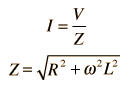Calculate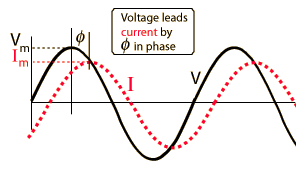Examine Inductor Resistor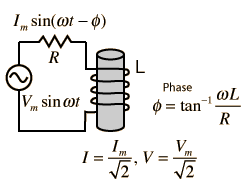Contribution tocomplex impedance Phasor diagram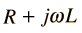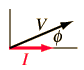You know that the voltage in an inductive circuit leads the current because the Lenz' law behavior resists the buildup of the current, and it takes a finite time for an imposed voltage to force the buildup of current to its maximum.

Index

Capacitance concepts

Inductance concepts

 HyperPhysics***** Electricity and Magnetism R Nave
Go Back

# RL Impedance

The frequency dependent impedance of an RL series circuit.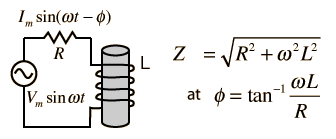For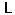= x10^ H = mH = microHenries
 at angular frequency ω = x10^ rad/s,
 frequency = x10^ Hz = kHz = MHz
 and resistance R = x10^ ohms = kohms = Megohms,

the impedance is

 Z = x10^ ohms = kohms = Megohms

 at phase φ = degrees.
Default values will be entered for unspecified parameters, but all component values can be changed. Click outside the box after entering data to initiate the calculation.
 AC behavior of RL circuit
Index

Capacitance concepts

Inductance concepts

 HyperPhysics***** Electricity and Magnetism R Nave
Go Back